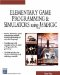# Round

This command rounds off a number with a decimal point (floating point number) to the nearest integer.

` x = Round(y) `

### Parameters

 x The rounded number y The number to be rounded off

### Notes

This is a useful command for sending values to the screen. For example, if you want the program user to know the distance between two objects, you can make numbers like 299.327 turn into 299 using the round command ”you do not need, nor necessarily want, the extra digits.

### Sample Code

` oworld = New World(); ocamera = New Camera(oworld); sphere = oworld.CreateSphere(50,50,50,25,25); ocamera.MoveBack(1200); sphere.Walk(); //Loop While(1) {  x = sphere.GetX();  xround = Round(x);  SetText(rounded  + xround +  not rounded + x); } `Elementary Game Programming & Simulations Using Jamagic (Charles River Media Game Development)
ISBN: 1584502614
EAN: 2147483647
Year: 2002
Pages: 105
Authors: Sergio Perez